Volume 12 Supplement 10

# Probabilistic reconstruction of the tumor progression process in gene regulatory networks in the presence of uncertainty

## Abstract

### Background

Accumulation of gene mutations in cells is known to be responsible for tumor progression, driving it from benign states to malignant states. However, previous studies have shown that the detailed sequence of gene mutations, or the steps in tumor progression, may vary from tumor to tumor, making it difficult to infer the exact path that a given type of tumor may have taken.

### Results

In this paper, we propose an effective probabilistic algorithm for reconstructing the tumor progression process based on partial knowledge of the underlying gene regulatory network and the steady state distribution of the gene expression values in a given tumor. We take the BNp (Boolean networks with pertubation) framework to model the gene regulatory networks. We assume that the true network is not exactly known but we are given an uncertainty class of networks that contains the true network. This network uncertainty class arises from our partial knowledge of the true network, typically represented as a set of local pathways that are embedded in the global network. Given the SSD of the cancerous network, we aim to simultaneously identify the true normal (healthy) network and the set of gene mutations that drove the network into the cancerous state. This is achieved by analyzing the effect of gene mutation on the SSD of a gene regulatory network. At each step, the proposed algorithm reduces the uncertainty class by keeping only those networks whose SSDs get close enough to the cancerous SSD as a result of additional gene mutation. These steps are repeated until we can find the best candidate for the true network and the most probable path of tumor progression.

### Conclusions

Simulation results based on both synthetic networks and networks constructed from actual pathway knowledge show that the proposed algorithm can identify the normal network and the actual path of tumor progression with high probability. The algorithm is also robust to model mismatch and allows us to control the trade-off between efficiency and accuracy.

## Background

The construction of gene regulatory networks is an extremely difficult problem owing to their complexity and the difficulty of obtaining the relevant time series data, in terms of sampling rate, measurement accuracy, and quantity. For instance, microarray data usually come in samples much too small for accurate inference, have a very low sampling rate relative to most cell signaling, measure average transcript across many cells, and are susceptible to many confounding factors which adversely affect the signal-to-noise ratio. In particular, for human cells, with data coming from patients, there are no time-course data and the data come from cells that have already undergone a sequence of mutations, so that the regulatory mechanisms of the original cell are no longer intact. Rather than depend on expression data, one can use known pathway information to construct regulatory relations and thereby develop an uncertainty class of networks whose regulatory dynamics are consistent with the pathway knowledge. An algorithm for doing this has been developed in the context of Boolean networks . If one could obtain wild-type time-course data, then one could reduce this uncertainty class by standard Boolean network inference methods. Given that in practice we usually only have access to stationary patient data and that the progression of mutations leading to the cancerous state has already occurred, we would like to use the available data to reduce the uncertainty class. In fact, since all we require is that we have an uncertainty class to begin with and wish to use the tumor data, from an algorithmic perspective it does not matter whether the uncertainty class arises from prior biological knowledge, wild-type data, or a combination of both. The proposed algorithm operates on the basis of probabilistic sequential fault-detection, which views regulatory alterations, such as gene mutations, as faults in the network wiring . It estimates the sequence of faults leading to the current (cancerous) regulatory structure, and from these estimates, a reduced uncertainty class for the original (healthy) network. By taking this approach the algorithm simultaneously accomplishes a dual purpose: network inference and fault detection.

The methodology is based on certain fundamental notions regarding cancer development, in particular, that the formation of a tumor is a complex process usually proceeding over a period of decades. Normal cells evolve into cells with increasingly neoplastic phenotypes. Tumor progression is driven by a sequence of randomly occurring mutations and epigenetic alterations of DNA that affect the genes controlling cell proliferation, survival, and other traits associated with malignant cell phenotype. To wit, tumor progression is a multi-step process of changes in the regulatory pathways. A set of pathways must be deregulated during the tumor progression until the tissue reaches a cancer state. A wide variety of normal adult human cell types can be transformed experimentally by perturbing five pathways . Certain normal human cells require a greater or lesser number of changes before they will become transformed. Moreover, the regulatory pathways can be altered in many different ways leading to the same cancer. For instance, studies on colon cancer show that the great majority (~ 90%) of colon carcinomas suffer inactivation of the APC gene on Chromosome 5q as an early step in this process, about 40% to 50% acquire a K-ras mutation, 50% to 70% show an LOH of Chromosome 17p involving p53, and about 60% show an LOH on Chromosome 18q. Most colon cancers will therefore begin with a Chromosome 5 alteration, but then will take alternative genetic paths on the road toward full-fledged malignancy .

In sum, although some common alterations may happen in tumor progression, different patients confront with different types of alterations during the progression, thereby making it important to find a way to identify mutations in order to apply appropriate intervention. Very little work has been done on the identification of genetic alterations (e.g. mutations) in cancer progression using network models. One such example is the work by Gerstung et al. , where they predicted cancer progression by applying a conjunctive Bayesian network, in which the order of gene mutations is extracted.

In this paper, we use the Boolean networks with perturbation (BNp) framework to model signaling pathways and ultimately predict the gene mutations that occurred during the tumor progression process. Boolean networks (BNs) have been used in a variety of other contexts and with different objectives in biological applications. Kauffman  proposed that the cell types are the attractors. He introduced randomization into the networks, in terms of environmental noise (random perturbation of individual genes) and mutation (not to be confused with the notion of mutation in cancer progression), which refers to changes in the wiring of the network. Random BNs and their characteristics have been extensively studied by Aldana et. al . In a random BN, the average function in-degrees are constant and function outputs are assigned randomly. Serra et al.  investigated the effects of perturbation in the context of random BNs by knocking out a single gene. Additionally, intervention in BNp has been also studied by Dougherty et al.  and Qian and Dougherty .

In this work, we focus on BNs with perturbation owing to their role in modeling gene regulatory networks, a key point being that their dynamics can be modeled as Markov chains, thereby facilitating the modeling of genetic alterations in signaling pathways by shifting the network steady state distribution (SSD) from the normal (healthy) SSD toward the cancerous SSD. Having this tool in one hand to model signaling pathways, and the cancerous SSD extracted from the malignant tissue (e.g., based on gene expression data) on the other hand, one can test all the possible alterations on the BN satisfying the pathway information to see which one makes the SSD of the altered BN as close to the cancerous SSD as possible. This allows one to track the sequence of mutations. However, there are two concerns for using BNps to model signaling pathways: (1) the network perturbation probability should be determined, and (2) signaling pathways provide us with incomplete information, which means that there may be too many BNs that satisfy the pathway information. The first issue can be mitigated by finding a good estimate of the perturbation probability. For example, inferring a BNp from a sequence of gene expression data has been studied in . In fact, the second issue is the main source of uncertainty in our problem. To the best of our knowledge, the paper by Layek et al.  is the only work that proposed a method to extract the BN underlying the normal tissue from a set of biological pathways. Although this paper introduced an elegant method for extracting the information needed for constructing Boolean networks from biological pathways, it yields a large number of networks since the available network knowledge is often incomplete and not enough to point out the true network. To address this issue, we define the notion of a family of Boolean networks, which is the set of all BNs that satisfy the constraints that are imposed by a given set of pathways. For instance, for a 6-gene signaling pathway, the resulting family can contain 212 networks, all of which satisfy the constraints imposed by the pathway.

As mentioned earlier, the main goal of this paper is twofold: (1) to infer the normal network underlying healthy cells from this family, and at the same time, (2) to find the set of alterations that have occurred during the cancer progression. Toward this goal, we propose a probabilistic sequential fault detection algorithm that can effectively identify the best candidates for the original normal (healthy) network and the accumulated gene mutations.

## Methods

### Boolean networks (BNs)

A Boolean network G (V, F) is defined by a set V = {x1, x2…,, x n } of binary variables, x i {0,1}, i = 1,…, n, and a list F = (f1, f2,…, f n ) of Boolean functions. The value of x i at time t + 1 is completely determined by a subset {x i 1, x i 2, … ,x i ki } V at time t via a Boolean function f i : {0, 1}ki {0,1}. Transitions are homogeneous in time and we have the update: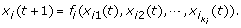(1)

Each x i represents the state (expression) of gene i, where x i = 1 and x i = 0 represent gene i being expressed and not expressed, respectively. It is commonplace to refer to x i as the i th gene. The list F of Boolean functions represents the rules of regulatory interactions between genes. That is, any given gene transforms its inputs (regulatory factors that bind to it) into an output, which is the state or expression of the gene itself. All genes are assumed to update synchronously in accordance with the functions assigned to them and this process is then repeated. At any time t, the state of the network is defined by a state vector x(t) = (x1(t), x2(t), ,x n (t)), called a gene activity profile (GAP). Given an initial state, a BN will eventually reach a set of states, called an attractor cycle, through which it will cycle endlessly. Each initial state corresponds to a unique attractor cycle and the set of states leading to a specific attractor cycle is known as the basin of attraction (BOA) of the attractor cycle.

A Boolean network with perturbation (BNp) is defined by allowing each gene to possess the possibility of randomly flipping its value with a positive probability p. Implicitly, we assume that there is an i.i.d. random perturbation vector γ = (γ1, γ2, …, γn), where γi {0, 1}, the i th gene flips if and only if γ i = 1, and p = P(γ i = 1) for i = 1, 2,…, n. If x(t) is the GAP at time t, then the next state x(t + 1) is either f(x(t)) with probability (1 – p)n or x(t) γ with probability 1 – (1 –p)n, where f is the multi-output function from the truth table and is component-wise addition modulo 2. Perturbation makes the corresponding Markov chain of a BNp irreducible and ergodic. Hence, the network possesses a steady state distribution, SSD(BNp), describing its long-run behavior. A BNp inherits the attractor structure from the original Boolean network without perturbation, the difference being that a random perturbation can cause a BNp to jump out of an attractor cycle, perhaps then transitioning to a different attractor cycle prior to another perturbation. If p is sufficiently small, then the SSD will reflect the attractor structure within the original network. We can derive the transition probability matrix (TPM) P if we know the truth table and the perturbation probability p for a BNp. The TPM of a BNp can be decomposed as: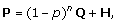(2)

where, Q is the TPM of the corresponding deterministic BN and H is a 2n × 2n matrix depending only on n and p .

We assume there exists a “normal” BN, denoted N normal , corresponding to a healthy wild-type phenotype, and a family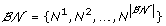of BNs possessing identical predictor sets as N normal such that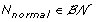. We refer to this familyas the “uncertainty class” relative to N normal .

Given a BN, we define an “alteration” to be a change in the rule structure (i.e., truth table). A “path” Path = {alt1 , alt2, …, alt M } is defined as a sequence of M alterations. From a modeling perspective, M denotes the number of alterations that have occurred during the tumor progression and alt j refers to the j th alteration. We assume that each alteration affects only a single gene and no two alterations in the same path affect the same gene. The result of applying a path of alterations to a Boolean network N is to produce an “altered network” [N; Path]. If we begin with a normal BN, N normal , and apply a “cancerous path”, Path c , we obtain a cancerous network N cancer =[N normal ; Path c ]. The following commutativity and associativity properties follow from the definition: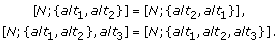(3)

Alterations in cancer progression are commonly gene mutations, and the accumulation of gene mutations is usually responsible for cancer. Gene mutation includes both oncogene activation and tumor suppressor gene deactivation, resulting in either continuous activation or deactivation of the corresponding genes. In the context of the BN model, such alteration in gene x i leads to permanently setting the boolean function to f i ≡ 1 or f i ≡ 0. We denote a gene mutation by a pair (i, k), which indicates that gene x i is stuck at k {0, 1}. For convenience, we define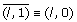and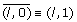. If a Boolean network N is altered by a mutation (i, k), this mutated BN is denoted as [N; {(i, k)}]. For example, for a 4-gene Boolean network N, [N; {(1,0), (4,1)}] refers to a mutated version of N, where gene x1 is permanently deactivated and gene x4 is permanently activated. In this case, in the regulatory truth-table, we will have f1 = 0 and f4 = 1 for every set of predictors. Gene mutation, also called “1-gene function perturbation”, has been studied . It should be noted that, physically, the order of mutations can make a difference in cancer progression, since alterations affect the regulatory structure, thereby affecting subsequent cancer progression. There is, however, no way to take this into account given that we only have steady-state data and no data on transient behavior. From a mathematical perspective, the commutativity in (3) means that a path is a set of alterations rather than a sequence of alterations; however, we employ the latter terminology owing to its commonplace usage.

Now the problem to be addressed in this paper can be stated as follows: Given a familyof Boolean networks, the steady state distribution (SSD) of the cancerous network, and the number of alterations M, what are the best candidates for N normal and Path c ? Searching for the best candidate for the normal network involves estimating the distance between altered networks and the cancerous network. Since the only available information about the cancerous network is its SSD, we need to define a distance measure between two networks based on their SSDs. Given two BNs with perturbation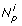and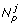, we compute their distance as follows: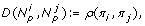(4)

where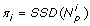,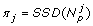, and ρij) is the Kullback-Leibler divergence (KL-divergence) between the SSDs π i and π j . This distance measure can be extended to BNs by first building the corresponding BNp for each BN using (2) and a given probability of perturbation p and then computing the distance between the resulting BNps. Without any ambiguity, in what follows, we use the same notation for a BN and the induced BNp for notational simplicity.

### Gene mutation effects

#### Effects of gene mutation in a BNp

In this section, we study the effect of a gene mutation (i, k) on the TPM of a BNp and its SSD. Gene mutations affect only the regulatory matrix Q in (2), where the mutation of each gene can be modeled as a multiplicative perturbation. Thus, for every mutation (i, k), we can find a corresponding transformation matrix Ti,k such that the TPM of the altered BNp is given by: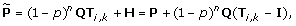(5)

where I is a 2n × 2n identity matrix. Based on this observation, we can easily prove the commutativity property shown in (3) (see Additional file 1 for the proof). According to the associative property shown in (3), a sequence of multiple gene mutations can be represented by a single transformation matrix, which is a product of the transformation matrices, each corresponding to a single gene mutation in the sequence. For example, the TPM of a BNp altered by a threefold mutation, [N; {(i1, k1), (i2, k2), (i3, k3)}], is given by: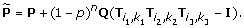The effect of rank-one perturbations in the TPM of a Markov chain on the SSD has been studied in the context of structural intervention in gene regulatory networks , and more generally in the framework of Markov chain perturbation theory . We can utilize these results to analyze the SSD of the altered BNp, whose TPM is given by (5).

In order to see how existing work on Markov chain perturbation can be used to analyze the effect of gene mutations on the SSD, consider two TPMs P and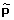that arise from the original network and the altered network, respectively. Let π andbe the SSDs of the two networks, such that πτP = πτ and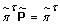. We can find the analytical expression of the change in SSD using the fundamental matrix Z = [IP + eπτ]–1, where e is an all-one column vector . The fundamental matrix Z exists for any ergodic Markov chain. Consider a rank-one perturbation, where the TPM of the perturbed Markov chain is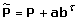, where a, b are two arbitrary vectors satisfying bτe = 0, and P is the TPM of the original Markov chain. In this case, it can be shown that  the following is true: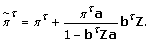(6)

Now, by representing the change of TPM due to a gene alteration as a sequence of rank-one perturbations, we can utilize (6) to predict the overall effect of the given mutation on the SSD of the network. To be more precise, suppose the BNp at hand undergoes a single mutation, (i, k). The transition probability matrixof the mutated BNp can be represented as follows: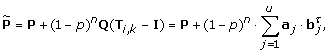(7)

for some vectors a j and b j satisfying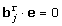, and a positive integer u ≤ 2n – 1. The proof can be found in Additional file 1. Based on (6) and (7), the SSD of the altered BNp can be iteratively calculated in at most 2n–1 iterations.

#### Example: effect of mutations in a 3-gene network

For illustration, let us consider a simple 3-gene BNp. Suppose the BNp is altered by (3,0), which means that the gene x3 is permanently deactivated such that x3 = 0. As a consequence, there cannot be any destination state in Q, which is the deterministic part of the TPM P in that arises from the regulatory structure of the BN, that corresponds to x3 = 1. Hence, if we let Q = [q1q8 ], where q j is the jth column in Q, the corresponding columns in Q should be shifted as follows: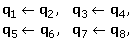where q j corresponds to the destination state with decimal representation j – 1, and q j q i means the jth column should be updated to q i + q j and the i th column to 0. Therefore, we get the following transformation matrix: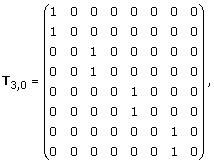and we have: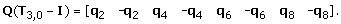(8)

Note that the rank of Q(T3,0I) is at most 2(n–1)= 4. Now, (8) can be decomposed as follows: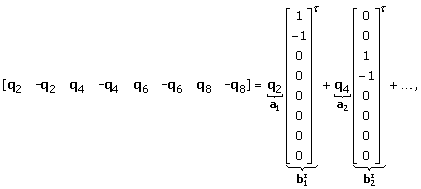(9)

wherefor all b i . From (9) and (5), we get: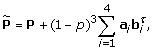(10)

which is in the form shown in (7). Now, by utilizing the result in (6), we can analytically compute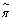through sequential rank-one perturbations as follows: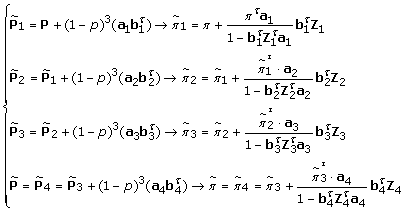(11)

where π and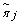are the SSD vectors for P and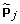, respectively, which satisfy πTP = πT and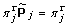. Z j are the corresponding fundamental matrices, as defined earlier.

### Overview of the proposed algorithm

Suppose we are given a family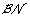of Boolean networks that contains the normal network N normal . Based on our definition, the cancerous network can be written as: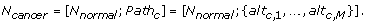Let SSD cancer denote the SSD of the cancerous network N cancer . We define the residual value for a given Boolean network N as: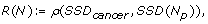(12)

where N p is the BNp with the regulatory matrix Q determined by the Boolean network N and the perturbation probability p. At each step, the algorithm alters the networks in the current family of networks through a single mutation. After the alterations, the algorithm keeps only those networks that lie within a certain distance from the cancerous network, where the distance is computed by (12). For the selected networks, the algorithm also keeps a record of the alterations that leads to these altered networks. Figure 1 provides an illustrative overview of the algorithm. Suppose that initially, the network family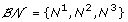contains three 3-gene networks. We assume that N1 is the true normal network N normal , and the cancerous network N cancer is obtained by taking the cancer progression path Path c = {(1, 0), (2,1)}, hence N cancer = [N normal ; {(1,0), (2,1)}]. Given the family, the SSD of the cancerous network SSD cancer , and the number of mutations (set to M = 2 in this example), the algorithm tries to identify the best candidates for the normal network N normal and the path Path c that may lead the original network into the cancerous network in two steps.

Initially, for each network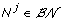, there can be 6 possible altered networks based on a single gene mutation. These altered networks are shown in the first row of Figure 1, in the middle plot. Among these networks, the algorithm only selects the networks whose SSDs are close to SSD cancer . Suppose we select the altered networks that are within β1-distance of cancerous network. The selected networks constitute a new (and reduced) uncertainty class of networks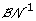. Next, each network incan be altered into 4 different networks based on an additional single gene mutation. These networks are shown in the second row of Figure 1, in the middle plot. Among these networks, the algorithm selects only those that are within β2-distance from the cancerous network, resulting in a further reduced uncertainty class of networks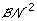. The familycontains the best candidates for the normal network and the cancerous path. For example,in Figure 1 contains two candidates (N1 and N2) for the normal network. For N1, the cancerous path {(1,0), (2,1)}, and equivalently, {(2,1), (1,0)}, may lead it to the cancerous network with the given steady state distribution SSD cancer . Similarly, N2 may be another candidate for the normal network, which may get close to the cancerous network also through the path {(1,0), (2,1)}. Note that the actual number of networks inand that inwill depend on the parameters β1 and β2, respectively.

### Details of the proposed algorithm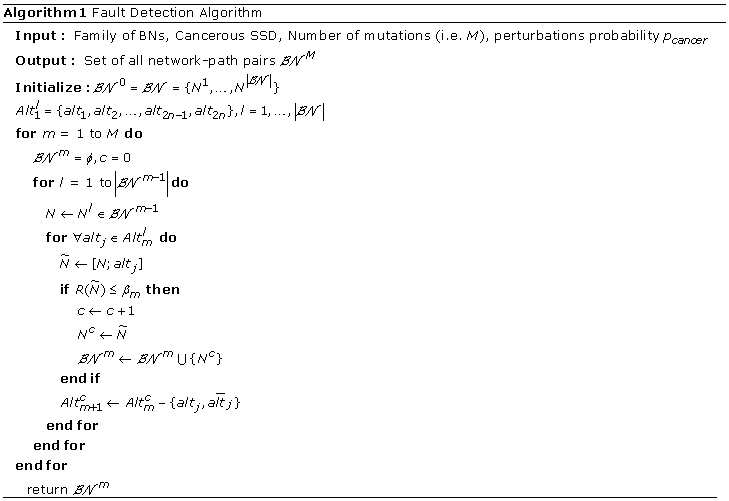The detailed procedure of the proposed algorithm is shown in Algorithm 1. At each step, one additional single gene mutation is considered. Therefore, to detect all M alterations that may have led the normal network into the cancerous network, the algorithm needs to go through M sequential steps. In the first step, we consider all possible single mutations of the form (i, k) for every network N in the family, which results in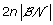altered networks. Among these networks, we select only those networks whose SSD can get close enough to SSD cancer , after M – 1 additional gene mutations. Based on this criterion, we select the network-mutation pairs, whose residual values (distance to the cancerous network measured based on SSD) are smaller than a threshold β1. In the second iteration we start with a new family of BNsthat contains the networks selected in the previous iteration. Since the gene that was mutated in the first step cannot go through another mutation, every network incan go through one of 2(n – 1) possible single gene mutations. Among these possible altered networks, we select only those networks whose residual values are smaller than a threshold β2. After repeating these steps M times, the final class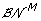will contain the best network-path pairs, where each pair consists of a candidate for the normal network and the cancerous path that may drive the given network into the cancerous state with the given SSD. In each iteration, the threshold β j can be used as a control parameter for trading between efficiency and accuracy. In general, we will have β1β2 ≥ … ≥ β M .

#### Performance metrics

In order to evaluate the performance of the algorithm, we define two metrics. The first metric is the probability that the algorithm will miss the true normal network N normal and the actual cancerous path Path c of length M: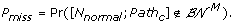(13)

We can estimate this probability as follows. Let us define: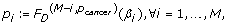(14)

where F D (Mi,pcancer)(d) is the cumulative distribution function (CDF) of the distance d between a BNp (with the perturbation probability p cancer ) and its altered version obtained by (M – i) mutations. Estimation of this CDF will be further discussed in the next section. Now, if we define: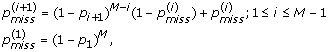(15)

we can show that: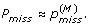(16)

The proof can be found in Additional file 1. The second metric to be used is the probability that the algorithm will not be able to detect any network within -distance of the true normal network N normal :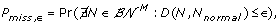(17)

These two metrics can be used to evaluate the accuracy of the proposed algorithm.

It would be also interesting to evaluate the computational complexity of the algorithm. When performing an exhaustive search, the total number of residual value computations that would be needed to find the final network familywould be: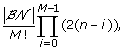which is exponential with respect to the number of mutations M.

Now, suppose that in the i th iteration of the proposed algorithm, α i % of the networks are selected (i.e.,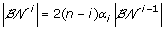) by controlling the parameter β i . For finding, our algorithm would need: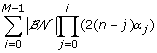(18)

residual value computations, where α0 = 1. A smaller β i will lead to a smaller α i , thereby reducing the overall complexity of the algorithm. However, this will also increase the probability of missing the true network, hence the parameters β j can be used to control the trade-off between computational efficiency and the prediction accuracy of the algorithm. As we can see from (18), the computational complexity of the proposed algorithm is polynomial with respect to the number of genes n (for a fixed M), while it is exponential with respect to the number of mutations M (for a fixed n). However, the parameters α j (j = 0, … , M – 1) allow one to trade between computational efficiency and prediction accuracy. As a result, the proposed algorithm can accurately reconstruct the cancer progression path in a much more efficient manner compared to the exhaustive search, as will be demonstrated in our simulation results.

### Cumulative distribution function of the distance between a random BNp and its mutated version

We estimate the CDF of the distance between a network and its altered version based on random BNs. We define a random Boolean network (RBN) as a BN: (1) whose gene predictors are randomly chosen such that every gene has k predictors, and (2) the truth table of every Boolean function f i follows an independent and identically distributed Bernoulli(pb) distribution, where p b is typically called the bias of the Boolean function f i . By allowing random perturbations with probability p in the RBN, we can obtain a random BNp (RBNp). First, we generate large number of RBNps with certain properties. Second, for each RBNp N p , we randomly introduce m mutations to obtain an altered network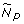, and measure their distance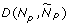. Based on these observations, we can estimate the CDF,where m is the number of single gene mutations and p is the perturbation probability in the RBNp.

## Results and discussion

### Estimating the CDF of the distance between networks

To execute the algorithm, we first estimated the CDF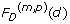of the distance d between an RBNp and its mutated copy. As with ensemble analysis in , we estimate these CDFs based on a large number of randomly generated networks with similar structural properties. The two most important parameters for generating random BNs are their bias probability p b and connectivity k. As described earlier, p b is the mean of the Bernoulli distribution used to randomly generate the predictor function for each gene in a BN, and k is the maximum number of input variables for each of these functions. We randomly generated 4,000 BNps with these properties. For each network, we introduced random gene mutations and computed the distance between the original BNp and the altered BNp. We used the MATLAB function KSDENSITY to find the CDF that best fits the observed distance distribution. We repeated the overall experiment for different numbers of genes n, different perturbation probabilities p, and different numbers of mutations m.

The estimated CDFs are shown in Figure 2, for several different parameters. Figure 2-(A) shows the estimation results for 6-gene networks for one or two gene mutations. We can see that the distance increases when we increase the number of mutations while keeping the probability of perturbation fixed. Similar behavior can be observed for 8-gene networks shown in Figure 2-(C). We can also see that the distance is generally larger for the 8-gene networks.

As we can see from Figure 2-(B), for 6-gene networks, increasing the perturbation probability from p = 0.001 to p = 0.01 decreases the distance. This is intuitive, since gene mutation (see (5)) only affects the regulatory part, which plays less important roles as the perturbation probability p increases. As a result, changing the regulatory structure of a BNp will have less significant effects when p is larger. Figure 2-(D) shows the results for 8-gene networks, which show similar tendencies.

### Performance of the algorithm on synthetic network families

We evaluated the performance of the proposed algorithm based on randomly generated families of Boolean networks through Monte Carlo simulations. All random networks in each of these families have identical structural properties (i.e., p b and k). In each family, one network whose Boolean functions are canalizing functions was selected as the true normal network N normal . A canalizing Boolean function is a function in which an input with a specific value determines the output of the function regardless of the other inputs. For instance, f(x1, x2) = x1ORx2 is a canalizing function, where x1 = 1 (and similarly, x2 = 1) will make the output f(x1,x2) = 1, regardless of the value of the other input variable. We randomly chose a path of length M and altered the normal network according to the given path to obtain the cancerous network. The steady state distribution (SSD) of the cancerous network was computed, to be used as an input for the proposed algorithm. Next, we used the proposed algorithm to find out whether it was able to infer the true normal network from a given family of networks and correctly predict the actual cancer progression path, when provided with the number of mutations M and the cancerous SSD. In our simulations, we used p cancer = 0.001, pb = 0.3, and k = 2. We considered 6-gene networks with M = 2 mutations and 8-gene networks with M = 3 mutations. For the case of 6-gene networks, we considered families of sizeand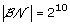. For the case of 8-gene networks, we considered families of size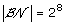. The algorithm was implemented using MATLAB 7.9.0 (R2009b), and all simulations have been performed on a desktop computer with 2.67GHz Intel Core i7 CPU and 12GB RAM. Each SSD computation took around 9.2 × 10–4 sec and 5.7 × 10–3 sec for n = 6 and n = 8, respectively.

Table 1 summarizes the results of applying our algorithm to 500 randomly generated network families, where each family contains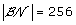6-gene networks and the normal network undergoes two gene mutations. The threshold β1 was chosen such that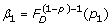for different values of p1. The second column in Table 1 shows the probability of missing the true network defined in (16). The third, fourth, and fifth columns show the empirically estimated probabilities. The sixth column shows the average number of networks in the final network family. The seventh column shows the average number of cancerous paths found in the final step, and the final column shows the average number of SSD computations needed for finding. As we can see in Table 1, increasing β1 (by controlling p1) decreases the probability of missing the true normal network but increases the number of networks (and the corresponding cancerous paths) included in the final network family. Furthermore, the number of SSD computations will increase if we use a larger β1 (by increasing p1). A similar trend can be also observed in Table 2, which summarizes the simulation results for 200 families with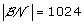6-gene networks.

We also evaluated the performance of the proposed algorithm based on randomly generated network families, each of which containsnetworks with 8-genes, with M = 3 gene mutations. The experimental results are summarized in Table 3. The first column in this table shows the probabilities p1 and p2 that were used to choose β1 and β2, using (14). The threshold β3 was set to β3 = 0.1. Table 3 shows that increasing the threshold values result in a higher probability of success (i.e., smaller probability of missing the true normal network) but also a higher computational cost, as we would expect. In practical situations, the actual perturbation probability p cancer may not be exactly known, in which case we would have to estimate the probability. To evaluate the robustness of the proposed algorithm, in the presence of model mismatch, we performed another set of simulations, whose results are summarized in Table 4. We used randomly generated network families, each with6-gene networks, and considered M = 2 mutations. As we can see in Table 4, there was no significant performance degradation when the perturbation probability p used by the algorithm was different from the true perturbation probability p cancer . The results for families withnetworks are summarized in Table 5, which show that the proposed algorithm is robust to model mismatch. Finally, Table 6 shows the results for network families with8-gene networks with M = 3 gene mutations, which also clearly shows the robustness of our algorithm.

### Performance on cancerous networks involving the p53 gene

Next, we evaluated the performance of the proposed algorithm based on a family of BNs constructed from pathways that involve the p 53 gene. Tumor suppressor gene p 53 has been extensively studied and it is known to be involved in various well-known biological pathways. It has been observed that p 53 is mutated in 30-50% of common human cancers . In fact, in the presence of DNA damage, a mutant p 53 may lead to the emergence of abnormal cells. Figure 3 shows the ATM-p53-Wip1–Mdm2 pathways that involve the tumor suppressor gene p53 .

These pathways operate in different ways depending on the context, determined by the presence (or absence) of a DNA damage event that results in DNA double-strand breaks. Here, we consider the case when DNA damage is present, which may lead to the development and progression of tumor. Under this context, we consider single and double mutations in the given pathways, where we focus on the mutation of p 53 and Mdm 2. Each gene alteration can be one of the three forms: mutation, amplification, or deletion. Sequencing data of 138 patients with glioblastoma, provided by TCGA, showed that 32% and 12% of the patients suffered from the alteration in the p 53 and Mdm 2 genes, respectively. Also among 316 patients with serous ovarian cancer, 96% suffered from the mutation of p 53. A similar study has revealed that about 26% of 216 patients with sarcoma have amplified Mdm 2. Mutation in p 53 and amplification in Mdm 2 have been also simultaneously observed in some cases. Based on these observations made in existing cancer studies, we consider the following types of alterations in our experiments: (p 53,0), (Mdm 2,1), and {(p 53, 0), (Mdm 2, 1)}, where p 53 is permanently deactivated and/or Mdm 2 is permanently activated. In a recent work , it has been shown that the pathways in Figure 3 do not uniquely determine the normal Boolean network N normal that governs healthy cells. We used the method proposed in  to enumerate all possible Boolean networks that satisfy the constraints imposed by the given pathways. Following , we constructed four Karnaugh maps, one for each gene in the given pathways. Karnaugh maps have been used in logic circuit design to simplify a given Boolean function and derive its minimal representation. In a Karnaugh Map , each position in the map (i.e., an element in a matrix) corresponds to a specific state (defined by the values of all genes in the network), such that neighboring states have unit Hamming distance. The value at each position indicates the value of a particular gene at the next time point, which is a Boolean function of the current state. The resulting maps are shown in Figure 4. In these tables, each line-segment, attached to a gene, shows the locations where that gene the takes value 1. The symbol X is used to indicate positions where the available pathway information was not enough for uniquely determining the table entries. These entries may take either 0 or 1 without violating the constraints. As a result, the given Karnaugh maps give rise to an uncertainty class of networksthat contains 212, where 12 is the number of entries in the given maps that cannot be uniquely determined. Since Mdm 2 is directly connected to three genes in Figure 3, we assume the connectivity to be k = 3, which is used to estimate the CDF of the distance between a random BNp and its altered version. The BN reported in  is assumed to be the true normal network N normal , as this network was shown to faithfully reproduce the experimentally observed behavior of the genes in published literature. We assumed p cancer to be 0.001. As in the previous section, we evaluated the performance of the proposed algorithm under two different cases: when we have a perfect estimate of the perturbation probability (p = p cancer ) and when there is a model mismatch (pp cancer ).

### Case-1: deactiviation of p53

We considered the alteration of the Boolean network reported in  through the permanent deactivation of p 53 (i.e. (p 53, 0)). We used our algorithm to detect the true normal network and the gene mutation. Table 7 shows the simulation result when the threshold was set to β1 = 0.05. The second column in the table shows the number of networks in the final network family, and the third column shows the total number of network-path pairs predicted by the algorithm. The fourth column shows the number of different paths in the predicted result. We also categorized the result of each experiment as a “success (S)” or a “failure (F)”, based on whether the final prediction contained the true network-path pair or not. As we can see, our algorithm was able to reduce the uncertainty class of networks without missing the true network for p = 0.001,0.003,0.005. For p = 0.007, the algorithm missed the true network, mainly because the perturbation probability was high enough to render the effects of the regulatory structure of the network relatively insignificant. Increasing β1 from 0.05 to 0.1 increases the number of network-path pairs included in the final prediction, thereby preventing the algorithm from missing the true network, as shown in Table 8. In terms of fault detection, the proposed algorithm performed very well. As shown in Table 7 and Table 8, the algorithm was able to correctly pinpoint the actual gene mutation out of 8 possible mutations, when it was successful.

### Case-2: amplification of Mdm2

Next, we altered the normal network by mutating Mdm2 such that it is amplified (i.e. (Mdm 2,1)). The results are summarized in Table 9 and Table 10 for β1 = 0.05 and β1 = 0.1, respectively. For β1, our algorithm was able to reduce the uncertainty class of networks without missing the true normal network for p = 0.001 and p = 0.003. When the perturbation probability became larger, the regulatory structure from the pathways was obscured, and the algorithm was not able to effectively reduce the uncertainty class (e.g., see Table 9, p = 0.005 and p = 0.007). By increasing β1 from 0.05 to 0.1, the algorithm could successfully reduce the uncertainty class for p = 0.005, as shown in Table 10.

### Case-3: simultaneous deactivation of p53 and amplification of Mdm2

Finally, we considered the case when p 53 was deactivated and Mdm 2 was amplified at the same time. Table 11 and Table 12 summarize the results of applying the proposed algorithm for the case of double gene mutations: (p 53,0) and (Mdm 2,1). As we would expect, the proposed algorithm did not perform well in this case, since introducing two gene mutations in a 4-gene network almost completely obscures the regulatory structure in the original normal network. In fact, the networks in the initial uncertainty classwill yield similar (or identical) SSDs once we mutate two genes. These results lead to an interesting insight into the expected performance of the proposed algorithm. As mentioned throughout the paper, the proposed algorithm aims to backtrace the set of gene mutations that has led to an (unknown) cancerous gene regulatory network with a given SSD. Suppose the number of mutations M is relatively small compared to the total number of genes n in the network (e.g., M/n ≈ 0). In such a case, the dynamics of the cancerous network would be largely governed by the regulatory mechanisms in the original healthy network. Even though it is theoretically possible that a few gene alterations lead to significant changes in the overall SSD, identifying these alterations would be still feasible since the regulatory structure of the original network would remain mostly intact. However, if the number of mutations gets larger (e.g., M/n ≈ 1), the activity of many genes would be “frozen”, either being permanently deactivated or permanently amplified, in which case the dynamics and the regulatory structure of the original network would be significantly lost. As a result, networks that originally have very distinct structures may yield similar SSDs as a result of the accrued mutations. In this case, it would be difficult for the algorithm to make predictions with high accuracy, since the available information would be too small to effectively cope with the present uncertainty.

## Conclusions

We proposed an effective probabilistic algorithm for reconstructing the tumor progression process. Given an uncertainty class of networks, which arises from our partial knowledge of the true gene regulatory network represented by biological pathways, and the steady state distribution of a cancerous network, the proposed algorithm tries to simultaneously infer the true gene regulatory network that underlies healthy cells and to predict the sequence of gene mutations that occurred during the tumor progression process. As demonstrated by our experiments, based on both randomly generated networks and realistic networks constructed from known biological pathways that involve the tumor suppressor gene p 53, our algorithm can effectively cope with the uncertainty present in gene regulatory networks and accurately infer the normal (healthy) network and the actual path of tumor progression with high probability. Furthermore, the proposed algorithm is robust to model mismatch and provides us with effective means for trading prediction accuracy for computational efficiency.

The computational complexity of the algorithm depends on the number of genes in the network, the number of mutations, and the number of networks in the initial uncertainty class, and increasing any of these numbers will increase the computational overhead. Based on the mathematical representation of Boolean networks, increasing the number of genes will exponentially increase the number of possible networks. However, this rapid increase does not necessarily mean that the size of the uncertainty class of networks that we need to deal with will increase at the same rate. For example, many of the mathematically possible networks may not be considered biologically viable, hence may be omitted in practice. Moreover, although the total number of states in a Boolean network with n genes is 2n, many states may be eliminated via state reduction, and the reduced network may consist of considerably fewer states . In fact, the whole idea of network reduction is relevant to the present problem, just as it is to determining control polices for gene regulatory networks, where computational intractability prohibits the design of control policies without constraining the network size . For example, even when the gene expression levels are restricted to be binary, a network with 15 genes, absent some form of state reduction, cannot be considered, because the size of the resulting transition probability matrix would be 215 × 215, making any kind of dynamic or control analysis intractable. Another possible way to reduce the complexity of the algorithm is to restrict the possible gene mutations via the use of prior knowledge. For example, we may restrict the possible mutant genes only to a smaller subset of genes that are known to be susceptible to mutation. Furthermore, prior knowledge concerning the expected type of mutation for a susceptible gene (e.g., “amplification” for oncogenes and “deactivation” for tumor suppressor genes) can be taken into account. Although we did not constrain the possible gene mutations nor applied any network reduction technique in this study, such modifications are fairly straightforward and may be used to enhance the overall computational efficiency of the proposed algorithm.

## References

1. Layek R, Datta A, Dougherty E: From biological pathways to regulatory networks. Mol. BioSyst 2011, 7: 843–851. 10.1039/c0mb00263a

2. Layek R, Datta A, Bittner M, Dougherty E: Cancer therapy design based on pathway logic. Bioinformatics 2011, 27(4):548. 10.1093/bioinformatics/btq703

3. Weinberg R: The biology of cancer. Garland Science New York; 2007.

4. Gerstung M, Baudis M, Moch H, Beerenwinkel N: Quantifying cancer progression with conjunctive Bayesian networks. Bioinformatics 2009, 25(21):2809. 10.1093/bioinformatics/btp505

5. Kauffman S: The origins of order: Self organization and selection in evolution. Oxford University Press, USA; 1993.

6. Aldana M, Coppersmith S, Kadanoff L: Boolean dynamics with random couplings. Perspectives and Problems in Nonlinear Science 2003, 23–89.

7. Serra R, Villani M, Semeria A: Genetic network models and statistical properties of gene expression data in knock-out experiments. Journal of theoretical biology 2004, 227: 149–157. 10.1016/j.jtbi.2003.10.018

8. Dougherty E, Pal R, Qian X, Bittner M, Datta A: Stationary and structural control in gene regulatory networks: basic concepts. International Journal of Systems Science 2010, 41: 5–16. 10.1080/00207720903144560

9. Qian X, Dougherty E: On the long-run sensitivity of probabilistic Boolean networks. Journal of theoretical biology 2009, 257(4):560–577. 10.1016/j.jtbi.2008.12.023

10. Marshall S, Yu L, Xiao Y, Dougherty E: Inference of a probabilistic Boolean network from a single observed temporal sequence. EURASIP Journal on Bioinformatics and Systems Biology 2007, 2007: 5.

11. Ivanov I, Simeonov P, Ghaffari N, Qian X, Dougherty E: Selection policy-induced reduction mappings for Boolean networks. Signal Processing, IEEE Transactions 2010, 58(9):4871–4882.

12. Hunter J: Stationary distributions and mean first passage times of perturbed Markov chains. Linear Algebra and its Applications 2005, 410: 217–243.

13. Schweitzer P: Perturbation theory and finite Markov chains. Journal of Applied Probability 1968, 5(2):401–413. 10.2307/3212261

14. Qian X, Dougherty E: Effect of function perturbation on the steady-state distribution of genetic regulatory networks: optimal structural intervention. IEEE Trans. Signal Process 2008, 56(10–1):4966–4976.

15. Kauffman S, Peterson C, Samuelsson B, Troein C: Genetic networks with canalyzing Boolean rules are always stable. Proceedings of the National Academy of Sciences of the United States of America 2004, 101(49):17102. 10.1073/pnas.0407783101

16. Shmulevich I, Dougherty E: Genomic Signal Processing (Princeton Series in Applied Mathematics). Princeton University Press; 2007.

17. Shmulevich I, Lahdesmaki H, Dougherty E, Astola J, Zhang W: The role of certain Post classes in Boolean network models of genetic networks. Proceedings of the National Academy of Sciences of the United States of America 2003, 100(19):10734. 10.1073/pnas.1534782100

18. Batchelor E, Loewer A, Lahav G: The ups and downs of p53: understanding protein dynamics in single cells. Nature Reviews Cancer 2009, 9(5):371–377. 10.1038/nrc2604

19. Karnaugh M: The map method for synthesis of combinational logic circuits. Trans. AIEE. pt. I 1953, 72(9):593–599.

20. Qian X, Ghaffari N, Ivanov I, Dougherty E: State reduction for network intervention in probabilistic Boolean networks. Bioinformatics 2010, 26(24):3098. 10.1093/bioinformatics/btq575

21. Ghaffari N, Ivanov I, Qian X, Dougherty E: A CoD-based reduction algorithm for designing stationary control policies on Boolean networks. Bioinformatics 2010, 26(12):1556. 10.1093/bioinformatics/btq225

## Acknowledgements

This work was supported by the W. M. Keck Foundation.

This article has been published as part of BMC Bioinformatics Volume 12 Supplement 10, 2011: Proceedings of the Eighth Annual MCBIOS Conference. Computational Biology and Bioinformatics for a New Decade. The full contents of the supplement are available online at http://www.biomedcentral.com/1471-2105/12?issue=S10.

## Author information

Authors

### Authors' contributions

Conceived and designed the experiments: MSE, ERD. Developed the algorithm and performed the experiments: MSE. Analyzed the data: MSE, ERD, BJY. Wrote the paper: MSE, ERD, BJY.

### Competing interests

The authors declare that they have no competing interests.

## Rights and permissions

Reprints and Permissions

Esfahani, M.S., Yoon, BJ. & Dougherty, E.R. Probabilistic reconstruction of the tumor progression process in gene regulatory networks in the presence of uncertainty. BMC Bioinformatics 12 (Suppl 10), S9 (2011). https://doi.org/10.1186/1471-2105-12-S10-S9